# How to calculate your Mortgage Payoff date with Extra Payments using Google Sheets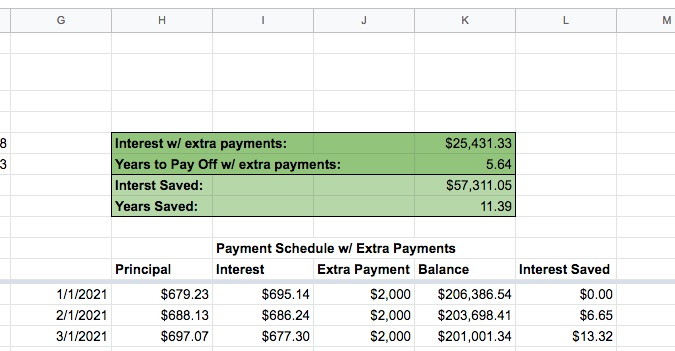Want an easy way to calculate how fast you can pay off your mortgage with extra payments? There are a lot of Mortgage Payoff Calculators out there but I decided to learn something new and create one myself using Google Sheets. After all it's just math? Can't be that hard... right? Right?

It took about an hour and some trial and error but in the end I created something great that will calculate how much quicker I can pay the loan off with extra payments and it also compares it to if I don't make any extra payments. I think it might be even more helpful to people than all the other calculators out there.

How do you do it you ask? Let's walk through it...

## First enter your basic loan info​

First thing to do is create a blank sheet and in the top right enter in the following info:
• Tracking start date: This is the date you want to start your calculations. It should be the first of the month. So in this case 12/1/2020.
• Loan Balance Remaining at start: This is your mortgage balance on that date
• Interest Rate
• Your regular monthly payment (principal and interest ONLY)
Like this: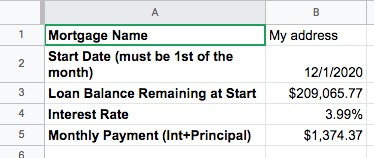Then below it you're going to make four headers for columns
• Month
• Principal
• Interest
• Balance
Like this: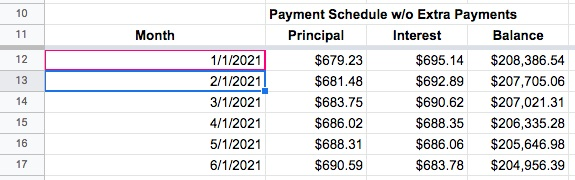## How to calculate mortgage amortization table​

You'll need to do this to figure out all of your payments if no extra payments are made. Plus it's pretty neat to see. To calculate your mortgage payments in an amortization table you need to do the following:
1. Take interest and multiply by remaining balance.
2. Divide by 12 to get your interest for that month.
3. Subtract that from your regular monthly payment to get the amount of principal you pay off for that month.
4. Repeat until paid off.
So now for the Sheets formulas....

The first row (A12 thru D12) will be a little different as it's the first row and it's pulling info from the top data that was entered. All other rows will pull data from the row above.

For the date column (A12) enter:
Code:
``=EDATE(B2, 1)``

The EDATE function advances from the start date (b2) 1 month to give you 1/1/2021 in this case. Why are we starting one month later and not with the date you entered at the top? Because you entered the value of your mortgage at that time and date and we are now calculating beyond that date.

For the interest column (C12) enter:
Code:
``=(\$B\$3*\$B\$4)/12``

Remember we calculate interest by taking the rate (B4) and multiplying it by the balance remaining (B3) and dividing that by 12 to get the interest that month. The () tells Google Sheets to do the calculation before dividing and the \$ tell it to keep the same source cells if we copy the code elsewhere.

For the balance column (D12) enter:
Code:
``=\$B\$3-(B12)``

B3 is the remaining balance entered at the top info. The \$ symbols makes it so that when we copy the code it keeps the same source cell (the loan balance remaining entered at top).

For the principal column (B12) enter:
Code:
``=\$B\$5-C12``

This takes B5 (your total monthly payment) and subtracts C12 which is your interest payment. The \$ symbols makes it so that when we copy the code it keeps the same source cell (the monthly payment entered at top).

### So that's the first row... it's different for the second as it'll be drawing info from the row above from now on...​

For the date column (A13) enter:
Code:
``==EDATE(A12, 1)``

This advances the date by one more month.

For the interest column (C13) enter:
Code:
``=(D12*\$B\$4)/12``

Same idea as last time we did interest but now we are drawing from the previous month's balance remaining which was D12 (the original starting balance minus principal paid last month).

For the balance column (D13) enter:
Code:
``=D12-B13``

This takes last month's balance and subtracts this month's principal payment.

For the principal column (B13) enter:
Code:
``=if(\$B\$5>D12,D12-C13,\$B\$5-C13)``

Now this one is a little different... and I learned the hard way. Usually to enter your principal payment you take your regular mortgage payment (B5) and subtract the calculated interest (C13). That's all fine and dandy when your balance is huge.... but what if your balance becomes less than your regular payment? It'll screw everything up that's what it'll do!

See here: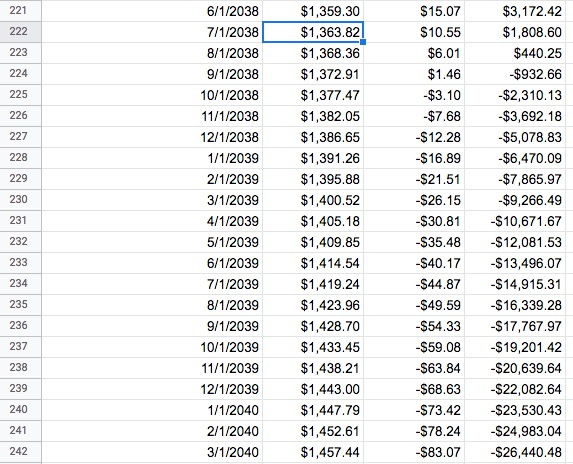See how the balance just races past zero and starts going into the negative until infinity? Yeah let's not have that happen.

We want to have something that checks if your remaining balance is less than you regular monthly payment... so:

Code:
``=if(\$B\$5>D12,D12,\$B\$5-C13)``

This says basically that IF your regular mortgage payment (B5) is greater than your current balance (D12) then the principal payment should just be your current balance (D12) BUT if it's LESS THAN the current balance it should be as normal and be your regular payment (B5) minus interest (C13)...

Much better: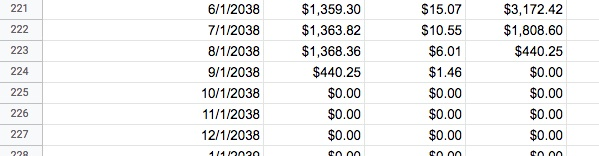Now it'll go to zero and not rush past. Of course if you get this close in real life you'd just ask the bank to give you the full payoff amount and just pay it off way before this when you're that low.### Now copy those rows downward...​

To copy the rows downward, hover your mouse in the bottom right of the cell: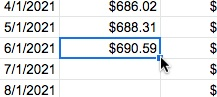You mouse pointer will turn into a crosshairs and just drag it all the way down and it'll replicated and autofill the cell downwards.

### Now you can calculate the total interest paid...​

To calculate your total interest paid let's create a cell that has the following:

Code:
``=SUM(C12:C500)``

Basically sums up the entire Interest column. In my case \$82,742.38. OUCH.

## Calculate time until your mortgage is paid off with the NPER function​

This is a new Google Sheet calculation I learned while figuring out how to do this. How does one figure out how many months or years until the mortgage is paid off? I COULD just scroll down until the date shows the balance paid off... but I want it shown in good ol' DATA form.

So let's enter this:

Code:
``=NPER(B4/12,-B5,B3)``

The NPER function calculates the number of payment periods for an investment based on constant-amount periodic payments and a constant interest rate.

The format is as such:

NPER(rate, payment_amount, present_value, future_value, end_or_beginning)

• rate - this is your APR or annual percentage rate... (B4) and you need to divide it by 12.
• payment_amount - This is your payment amount (B5) but it needs to be negative.
• present_value - The current loan balance remaining (B3)
• future_value - This is optional - we'll leave off
• end_or_beginning - This is optional - we'll leave off
That brings my payoff duration to 212.3210289 months. Use these button to round decimal places: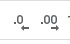So 212.32 months. Divide by 12 to get years which is 17.69. Scrolling down my spreadsheet brings it to around August 2038. Ouch. Let's change that.

## Let's start paying this mortgage off early!​

Go ahead and copy all of the Columns A thru D over to the right in your Google Sheet. But we're going to make a bunch of changes.

First off when you copy the cells over you may need to make some adjustments in your source cells. Go ahead and change that. But we're also going to add an "Extra Payment" column and an "Interest Saved" Column.

We're also going to add at the top to enter data an "Extra Payment" cell.

In this cell you're going to enter how much more each month you're going to a pay each month.

The extra payment column simply draws from this cell and populates it down so let's enter this data into the column:

Code:
``=\$E\$3``

(E3) is my extra payment cell. Why have this extra payment column at all? Because this is for the estimating Google Sheet. We're also going to create an ACTUAL section on this sheet that will track
how much you are saving as you pay as you go. And let's be real.... you're probably not always going to pay the exact same extra each month.But that is probably for another post.

The math is a little different now though for the Balance column (K) in this case. It is now:

Code:
``=\$B\$3-(H12+J12)``

on the top cell and:

Code:
``=K12-(H13+J13)``

on the cell beneath it.

It now says that the current balance is last months balance (K12) minus your regular principal payment (H13) and your extra payment (J13).

The interest saved column is simply the difference between the interest in the column with no extra payments and the column with extra payments:

Code:
``=C13-I13``

Now at the top we can now calculate the same way as before your total interest with extra payments and it's difference without extra payments:

Now of course autofill the cells downward as we did before.The NPER code is slightly different as we need to add the extra payment before we multiply:

Code:
``=NPER(B4/12,-(B5+E3),B3)``

Then simply calculate the difference between the two NPER calculations to display the time saved.

Voila! You did it!

Here's the template if you need it:

I hope you learned something on how to do something new in Google Sheets! But even more important I hope this gets you jazzed up and excited about paying down your mortgage MUCH earlier than with regular payments.Started The Mortgage Payoff Club in 2015 after being inspired by Dave Ramsey's "Total Money Makeover", Elizabeth Warren's "All Your Worth", and the blog Budgets are Sexy. Life got in the way and I haven't been able to be as aggressive as I'd like in paying it off over the years but a total of over \$29,000 has been paid down so far and I've saved \$37k in interest. If I continue at my current payoff rate my estimated payoff date is 2/1/2029. I'm going to try and get that lower. (original payoff date was 6/1/2042!!)

There are no comments to display.

Author
cdub
Views
738
Last update